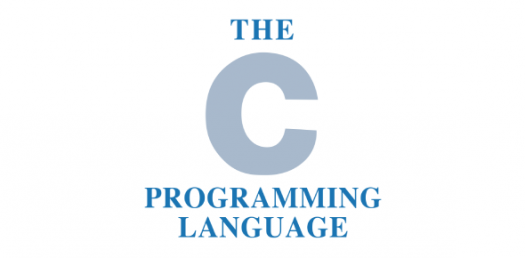# C Language Programming Trivia Questions! Quiz

25 Questions | Total Attempts: 44SettingsAre you ready to tackle this C language programming trivia questions? How much do you think you know about C Language programming? This quiz allows you to test your knowledge, not to mention your full comprehension of the subject. If you call your self a C Language Programming guru, take it up now and find out how good you are. All the very beast as you attempt this quiz.

Related Topics
• 1.
What punctuation must use to end the code C/C++ expression statement?
• A.

. (dot)

• B.

; (semi-colon)

• C.

: (colon)

• D.

‘ (single quote)

• 2.
What symbol must use to signal the beginning and end of code blocks or compound statements?
• A.

{ }

• B.

-> and

• C.

BEGIN and END

• D.

( and )

• 3.
Which of the following is a correct comment for comment in C programs?
• A.

• B.

** Comment **

• C.

/* Comment */

• D.

{ Comment }

• 4.
What value will return to the operating system upon the successful completion of a program?
• A.

- 1

• B.

1

• C.

0

• D.

Programs do not return a value

• 5.
Which one of the following is the correct operator to compare two variables?
• A.

: =

• B.

=

• C.

Equal

• D.

= =

• 6.
The directives for the preprocessors begin with
• A.

Ampersand symbol (&)

• B.

Two Slashes (//)

• C.

Number Sign (#)

• D.

Less than symbol (

• 7.
Which one of the following is the boolean operator for logical and?
• A.

&

• B.

& &

• C.

|

• D.

| &

• 8.
“C” was primarily developed as a
• A.

Systems Programing Language

• B.

General Purpose Language

• C.

Data Processing Language

• D.

None

• 9.
“C” is a
• A.

High Level Language

• B.

Low Level Language

• C.

High Level Language with some low level features

• D.

Low level language with some high level features

• 10.
Most suitable option for  “C” literal is
• A.

A string

• B.

A string constant

• C.

A character

• D.

An alphabet

• 11.
Length of the string “Correct” is
• A.

7

• B.

8

• C.

6

• D.

Implementation dependent

• 12.
Choose the correct statement
• A.

• B.

An identifier may end with an underscore

• C.

IF is a valid identifier

• D.

All

• 13.
The “const” feature can be applied to
• A.

An identifier

• B.

An array

• C.

An array argument

• D.

All

• 14.
Pick the operator that associate from the left
• A.

+

• B.

,

• C.

• D.

All

• 15.
Choose the correct statement
• A.

Constant expressions are evaluated at compile time

• B.

String constants can be concatenated at compile time

• C.

Size of array must be known at compile time

• D.

All

• 16.
Pick the operator that associate from the right
• A.

? :

• B.

+ =

• C.

=

• D.

All

• 17.
• A.

It is a unary operator

• B.

It cannot be applied to an expression

• C.

It associates from the right

• D.

All

• 18.
C Supports Structured Programming Technique.
• A.

True

• B.

False

• 19.
Which of the following is not a valid variable name declaration?
• A.

Int a3;

• B.

Int 3a;

• C.

int A3

• D.

None of the mentioned

• 20.
All keywords in C are in
• A.

LowerCase letters

• B.

UpperCase letters

• C.

CamelCase letters

• D.

None

• 21.
Which of the following is true for variable names in C?
• A.

They can contain alphanumeric characters as well as special characters

• B.

It is not an error to declare a variable to be one of the keywords(like goto, static)

• C.

• D.

Variable can be of any length

• 22.
Comment on the output of this C code?
1. #include <stdio.h>
2. int main()
3. {
4. int a = {1, 2, 3, 4, 5};
5. int i;
6. for (i = 0; i < 5; i++)
7. if ((char)a[i] == '5')
8. printf("%d\n", a[i]);
9. else
10. printf("FAIL\n");
• A.

The compiler will flag an error

• B.

Program will compile and print the output 5

• C.

Program will compile and print the ASCII value of 5

• D.

Program will compile and print FAIL for 5 times

• 23.
An _______ is Set of Same type of locations.
• 24.
C is case sensitive language.
• A.

True

• B.

False